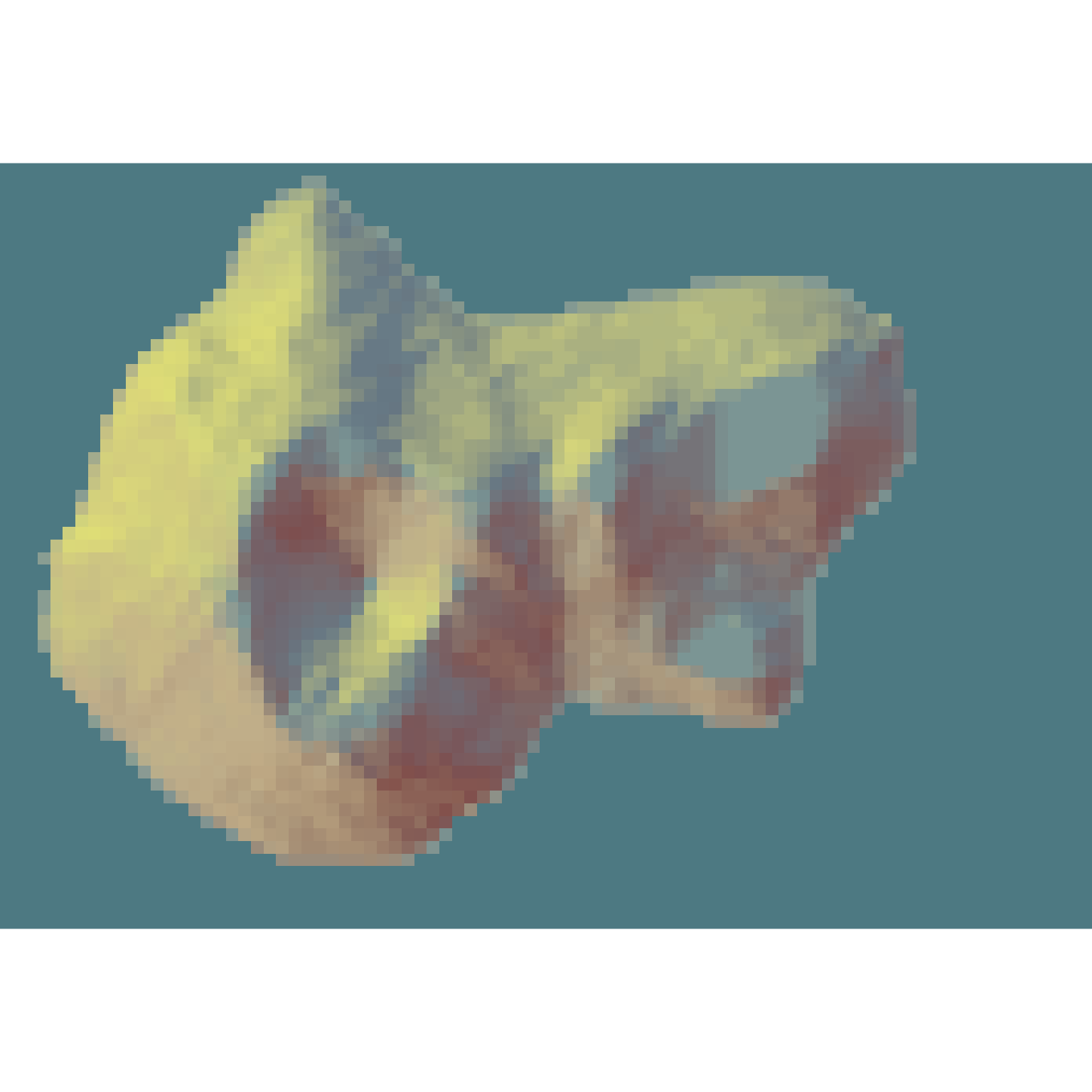Detects bodies of water (of a user-defined minimum size) within an elevation matrix.

detect_water(
heightmap,
zscale = 1,
cutoff = 0.999,
min_area = length(heightmap)/400,
max_height = NULL,
normalvectors = NULL,
keep_groups = FALSE,
progbar = FALSE
)

## Arguments

heightmap

A two-dimensional matrix, where each entry in the matrix is the elevation at that point. All grid points are assumed to be evenly spaced.

zscale

Default 1. The ratio between the x and y spacing (which are assumed to be equal) and the z axis. For example, if the elevation levels are in units of 1 meter and the grid values are separated by 10 meters, zscale would be 10.

cutoff

Default 0.999. The lower limit of the z-component of the unit normal vector to be classified as water.

min_area

Default length(heightmap)/400. Minimum area (in units of the height matrix x and y spacing) to be considered a body of water.

max_height

Default NULL. If passed, this number will specify the maximum height a point can be considered to be water.

normalvectors

Default NULL. Pre-computed array of normal vectors from the calculate_normal function. Supplying this will speed up water detection.

keep_groups

Default FALSE. If TRUE, the matrix returned will retain the numbered grouping information.

progbar

Default FALSE. If TRUE, turns on progress bar.

## Value

Matrix indicating whether water was detected at that point. 1 indicates water, 0 indicates no water.

## Examples

library(magrittr)
#Here we even out a portion of the volcano dataset to simulate water:
island_volcano = volcano
island_volcano[island_volcano < mean(island_volcano)] = mean(island_volcano)

#Setting a minimum area avoids classifying small flat areas as water:
island_volcano %>%